The International Information Center for Geotechnical Engineers

# Prefabricated Vertical Drains

Features

PVDs have a channeled or studded plastic core wrapped with a geotextile. The plastic core functions as support for the filter fabric, and provides longitudinal flow paths along the drain length. It also provides resistance to longitudinal stretching as well as buckling of the drain. The drain jacket acts as a filter to limit the passage of fine grained soil into the core area. It also functions to prevent closure of the internal water flow paths under lateral soil pressure.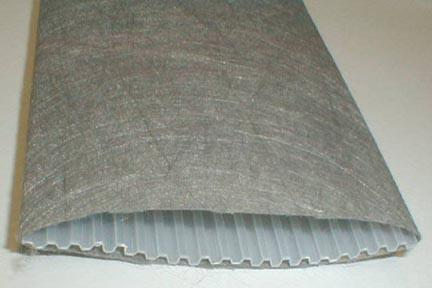[C] Layfield Wick Drain Example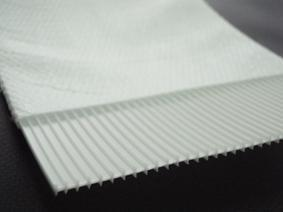[D] Geosupply Wick Drain Example

Light-weight drains have a width to thickness ratio of 30-35. It is desirable for the surface area, which will permit seepage into the drain, to be 0.2-0.3 in2 (150-200 mm2) per 0.4 in (1 mm) length. The wick drains should be installed with a center to center spacing of 3 to 8 ft . This will be discussed more in the design parameters section.

Equivalent Diameter

Wick drains have an oblong shape, but the available theories of radial consolidation have been derived for drains having a circular shape, often taken as equal to the nominal diameter of the sand drain.  Fellenius (1977) suggested that the equivalent cylindrical diameter of a sand drain is the nominal diameter of the sand drain multiplied by the porosity of the sand in the drain . The porosity of loose, free-draining sand can range from 0.4 to 0.5. This would result an equivalent cylindrical diameter of a sand drain of approximately half of the nominal diameter. In order to apply these theories to the design of field installations of wick drains, the equivalent diameter of an oblong shape is needed. Many equations have been suggested for computing the equivalent diameter, but each computation yields different results because they were derived under different assumptions.

The equations predicting the progress of consolidation around vertical drains assume a circular cross section for both the drain itself and the area the drain influences (Barron 1948).

Common wick drains have a plastic core surrounded by a geotextile: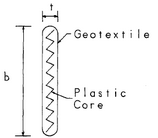[E] Cross-Section of PVD

Hansbo (1979) used a finite-element analysis and reported that the equivalent diameter of a drain is given as: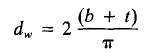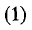Where:

dw = equivalent diameter of the drain
b = width of the prefabricated drain
t = drain thickness

Rixner et al. (1986) suggests the following few equations: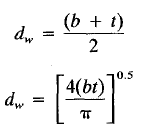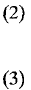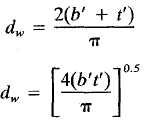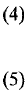Where:

2(b+ t) is used for free or open drain circumference

b* t is used for free or open drain cross section

b and t are reduced dimensions to account for clogging of the drain surface

In an independent evaluation by Richard P. Long and Alvaro Covo (1994), an electrical analog is used to define an equivalent diameter via evaluation of the previously listed equations’ varying results. A Sunshine Analog Field Plotter and electrically conducting paper were used. This technique uses the analogies of electrical potential to hydraulic head and electrical current to flow of water .

The procedure is represented as steady-state water flow for a model of ¼ of the drain cross section. A quarter of the cross section is used to eliminate repetition since the drain has two axes of symmetry. The model, seen below, is not applicable in the immediate vicinity of the drain at short times after consolidation begins. When water flows through a circular drain, rather than the supposed oblong profile, a shape factor for the flow net should be used .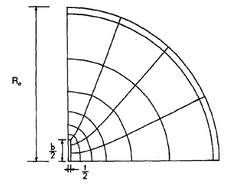[F] Flow Net for Flow to Oblong Drain from Circular Source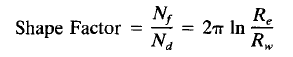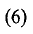Re = outside radius of the area

Rw = radius of central well

Nf = number of flow paths

Nd = number of head drops

Re, b, and t were varied throughout the Foott & Ladd (1981) study, and for each combination of values, a flow net was constructed using an analog field plotter. Using the shape factor and a summarization of the field plotter results, an equivalent diameter was determined.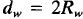The results of the analog modeling are plotted below. The ratio of the computed equivalent diameter of the drain, dw, to the width, b, is plotted as the vertical axis and the ratio of the thickness of the drain, t, to width of drain, b, is plotted as the horizontal axis. The ratio of the thickness to width, t/b, in the analog model varied from 0 to 1.0, which includes all possible combinations of drain thickness and width. The results are represented by using an x and a straight line of least squares fit is shown.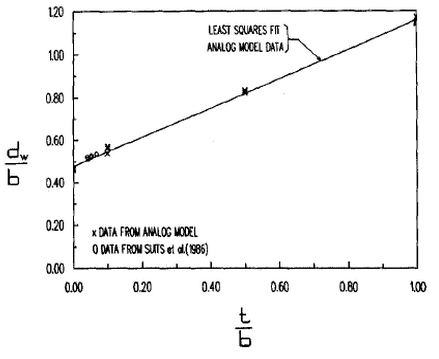[G] Plot of Equivalent-Drain-Diameter to Width-of-Drain Ration, d/b versus Thickness-of-Drain to Width-of-Drain Ration, t/v

Also on Fig. 3 are circles representing Suits et al. (1986) results from an experimental evaluation of equivalent diameters of drains.

Suits et al. method requires determining the coefficient of consolidation for remolded soil samples by standard laboratory tests, then consolidating the same soils using wick drains. The soils used for this experiment include, but are not limited to clay, organic silty clay, and peat. The reported values are approximately equal to the prediction from the linear least squares model .

Assuming negligible resistance to water flow, a unique value for dw, based on the model data for drain thickness and width, may be calculated. Equation (7) indicates that for t = b, dw = 1.2b, approximately the diameter of a circle with area b2. Equation (7) yields an appropriate value of the equivalent diameter of the drain for all t/b.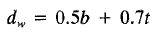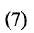Values calculated from equations (1), (2), (3), and (7), and are combined with average experimental values from Suits et al. (1986) are shown in Table 1. The NYLEX and AMER-DRAIN were tested with clay only, but all over drain types were tested with each soil. Equations (2) and (7) report values similar to the experimental values. Whereas equation (1) values are consistently larger, and equation (3) values are smaller. Equations (2) and (7) seem to yield regularly agreeable values, however when b = t, the two methods yield results that vary by 20% .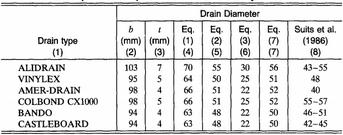[H] Comparison of Equivalent Drain Diameters by Various Methods

In summary, the equivalent diameter is essential for proper interpretation of field data to assess field conditions. The equivalent diameter of the shape of prefabricated drains was determined using an electrical analog field plotter. Values determined by this method agree with experimental values of Suits et al. (1986). Equivalent diameters of presently available prefabricated drains can be computed with reasonable accuracy from either equation (2) or (7), but (7) yields a more accurate value for all t/b.